summary of site-wide JavaScript functionalityUnited States-English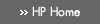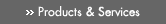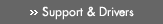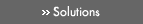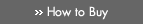» Contact HPSearch:More optionsManualTechnical documentation - EnglishAll of HP USHP-UX Reference > R# remainder(3M)

HP-UX 11i Version 3: February 2007»

## Technical documentation» Feedback## » Index## NAME

remainder(), remainderf(), remainderl(), remainderw(), remainderq() — remainder functions

## SYNOPSIS

#include <math.h>

double remainder(double x, double y);

### HP Integrity Server Only

float remainderf(float x, float y);

long double remainderl(long double x, long double y);

extended remainderw(extended x, extended y);

## DESCRIPTION

The remainder() function returns the floating-point remainder r = x - ny when y is a nonzero number. The value n is the integral value nearest the exact value x/y; when | n - x/y | = ½, the value n is chosen to be even.

The remainder() function is independent of the rounding mode.

### Integrity Server Only

remainderf() is a float version of remainder(); it takes float arguments and returns a float result.

remainderl() is a long double version of remainder(); it takes long double arguments and returns a long double result.

remainderw() is an extended version of remainder(); it takes extended arguments and returns an extended result.

remainderq() is equivalent to remainderl() on HP-UX systems.

## USAGE

To use these functions, compile either with the default -Ae option or with the -Aa and -D_HPUX_SOURCE options.

To use (for Integrity servers) remainderw() or remainderq(), compile also with the -fpwidetypes option.

Make sure your program includes <math.h>. Link in the math library by specifying -lm on the compiler or linker command line.

## RETURN VALUE

If y is ±INFINITY and x is not ±INFINITY, remainder() returns x.

If x is ±zero and y is a nonzero number, remainder() returns x.

If x or y is NaN, remainder() returns NaN.

If y is zero, remainder() returns NaN and raises the invalid exception.

If x is ±INFINITY, remainder() returns NaN and raises the invalid exception.

## ERRORS

If y is zero or x is infinite, remainder() sets errno to [EDOM].

### Integrity Server Only

HP-UX libm functions on Integrity servers do not set errno by default. For errno setting, compile with the +Olibmerrno option and the (default) +Olibcalls option.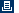Printable versionPrivacy statement Using this site means you accept its terms Feedback to webmaster© 1983-2007 Hewlett-Packard Development Company, L.P.